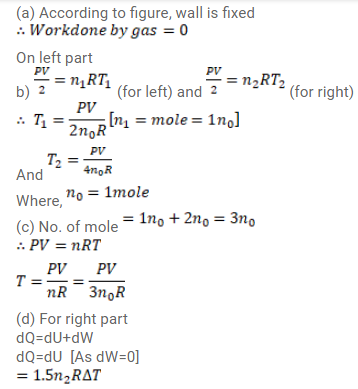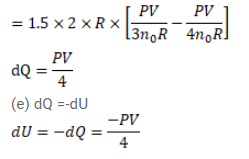# An adiabatic vessel of total volume V is divided into two equal partsQuestion:

An adiabatic vessel of total volume $\mathrm{V}$ is divided into two equal parts by a conducting separator. The separator is fixed in this position. The part on the contains one mole of an ideal gas $(U=1.5 n R T)$ and the part on the right contains two moles of the same gas. Initially, the pressure on each side is p. The system is left for sufficient time so that a steady state is reached. Find

(a) the work done by the gas in the left part during the process,

(b) the temperature on the two sides in the beginning,

(c) the final common temperature reached by the gases,

(b) the heat given to the gas in the right part and

(e) the increase in the internal energy of the gas is the left part.

Solution: